﻿ MotionDesigner Reference & User Interface > SEGMENT EDITOR > Segment Editor: Segment-Range

# Segment Editor: Segment-Range

### Segment Editor :  Segment-Range

Many Traditional Motion-Laws have Segment-Range parameters.

The Segment-Range parameters specify the phases at which to start and end the mathematical-function of the motion-law.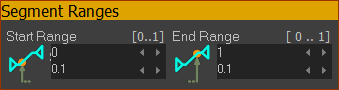Default Segment Ranges Start-Range ≥ 0 End-Range ≤ 1 0 ≤ Start Range < End Range ≤ 1

Segment-Range parameters do NOT change the Segment-Width.

One application of Segment-Range is to design Asymmetrical Motions - see below.

#### Example:

The images below show the acceleration graph of a Modified Sinusoid motion-law with different Segment-Range parameter values.

Note: the Segment-Width does not change.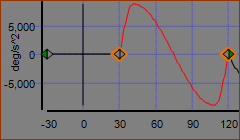1) Standard Mod Sine

1) Modified Sinusoid Motion-Law.

 Segment-Width = 120º-30º = 90º •Start-Range = 0.0 •End-Range = 1.0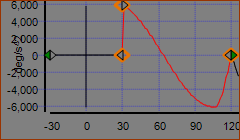2) Start Range = 0.2 ; End-Range = 1

2)  Modified-Sinusoid - 20% clipped from its Start.

 Segment-Width = 120º-30º = 90º •Start-Range = 0.2 - to remove 0 - 20% from the start of the Modified-Sinusoid mathematical function •End-Range = 1.0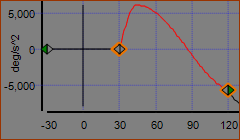Start Range = 0.0 ; End-Range = 0.8

Modified-Sinusoid - 20% clipped from the End.

 Segment-Width = 120º-30º = 90º •Start-Range = 0.0 •End-Range = 0.8 - to remove the 20% from the end of the Modified-Sinusoid mathematical function

### Asymmetric Motion

You can use an Asymmetric Motion to accelerate rapidly and decelerate more gradually, or vice versa.

In MotionDesigner, Asymmetric motion-laws combine two segments.

In the image below, there is a Rise and Return motion. Usually, you would need two segments.

However, there are three segments.

Segments 1 (0-60º) and Segment 2 (60º-180º) combine to give an asymmetric Mod-Sine. The crossover is at 60º.

Segment 3 (180º-360º) is a symmetric Mod-Sine. The crossover is at 270º the mid-point.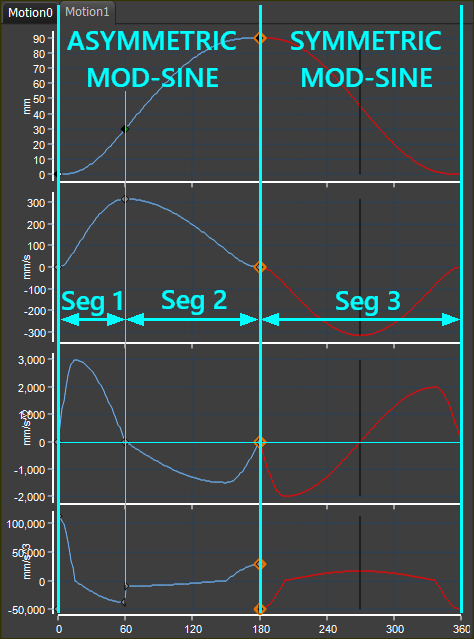Aysymmetric Mod-Sine   |   Symmetric Mod-Sine

Asymmetrical Mod-Sine Segments :

Segment 1

Machine angle : ( of total Asymmetric motion-law )

Displacement : ( of total Asymmetric Displacement )

Start-Range = 0 ; End Range = 0.5

Segment 2

Machine angle : ( of total Asymmetric motion-law )

Displacement : ( of total Asymmetric Displacement )

Start-Range = 0.5 ; End Range = 1.0

Characteristics Values: Asymmetric and Symmetric Compared.

 Velocity Coefficient : Acceleration Coefficient : Deceleration Coefficient : Jerk at Crossover :